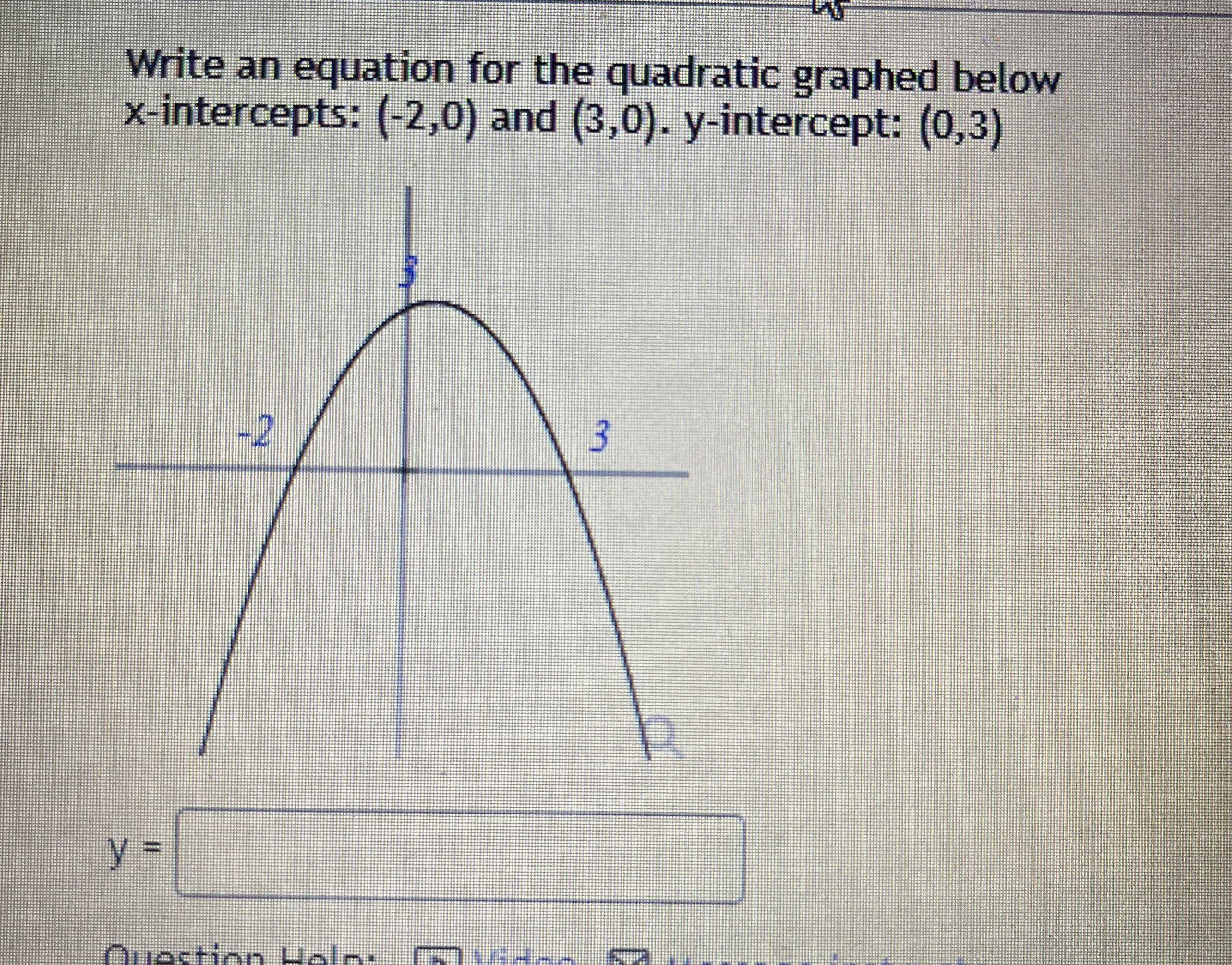### ¿Todavía tienes preguntas de matemáticas?

Pregunte a nuestros tutores expertos
Algebra
PreguntaWrite an equation for the quadratic graphed below

$$x$$ -intercepts: $$( - 2,0 )$$ and $$( 3,0 ) . y$$ -intercept: $$( 0,3 )$$

$$y = \frac{- x^2}{3} + \frac{x}{2} + 3$$